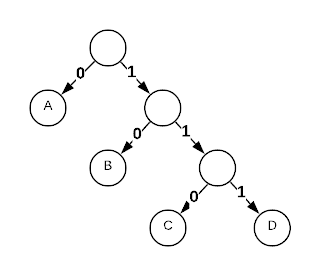# Huffman Codes

Under Construction: Consider this content a preview of the real thing which is coming soon (hopefully).

Prerequisites: Heap, Binary Tree

Huffman codes are a popular means of lossless compression. The general idea is to assign binary codes to each character in a file. Compression consists of converting each character to it’s corresponding code (which is hopefully much shorter). The file is decompressed by preforming the process in reverse.

Before jumping right into Huffman codes, it’s advantageous to understand codes in general. First, consider fixed length codes. A simplistic code for the first four characters of the alphabet is shown below.

Symbol Code
A 00
B 01
C 10
D 11

The string ABCD in it’s uncompressed ASCII form is 01000001010000100100001101000100 (32 bits). It is thus compressed as 00011011 (8 bits). Decompressing is as easy as reading two bits at a time and converting them to the corresponding letter.

Another type of code is a variable length code. As the name implies, each code can have a different length. If shorter codes are used for the most frequently used symbols, the resulting compressed message is much smaller. Consider the code defined below.

Symbol Code
A 0
B 01
C 10
D 1

The string ABAD in ASCII is 01000001010000100100000101000100 (32 bits). The compressed form is a bit shorter than it’s fixed length equivalent: 00101 (5 bits). There is one glaring problem with this code: it’s impossible to decode. The first three characters 001 could be decoded as either AAD or AB.

Prefix-free codes make variable length codes useable. The concept is that no code is a prefix of another code. Consider the prefix-free code defined below.

Symbol Code
A 0
B 10
C 110
D 111

Using this code, the string ABAD is encoded as 0100111 (7 bits). Decoding is now possible by using a binary tree. Simply follow the links in the tree and the first symbol encountered is the correct one.The trick to creating an efficient prefix-free code is to assign the shortest codes to the most frequently used symbols and the longest codes to the least frequently used symbols. Consider the symbol distribution below.

Symbol Code Freq
A 0 60%
B 10 25%
C 110 10%
D 111 5%

To find the average bits per symbol, sum the product of each code length by its frequency as shown below.

$(1*.6)+(2*.25)+(3*.1)+(3*.05) = 1.55$

The Huffman code algorithm produces a binary tree that results in the minimum average bits per symbol for a given distribution of symbols.

## Applications

• Geneticist calculate the frequencies of As, Cs, Gs, and Ts in human DNA to generate an efficient code
• MP3 encoding computes symbol frequencies before compression

## Asymptotic Complexity

$O(n\log n)$

## Pseudo Code

freqs = array of (character, frequency) tuples
H = min heap of (character, frequency) tuples, key=frequency

for each freq in freqs:
node
node->zero = NULL
node->one = NULL
node->frequency = freq->frequency
node->character = freq->character

H->insert node

while H->size > 0:
left = H->extract
right = h->extract
if(right == NULL):
return left
newNode
newNode->zero = left
newNode->one = right
newNode->frequency = left->frequency + right->frequency
newNode->character = NULL // Only leaf nodes get a character
H->insert newNode


Full Repo

Relevant Files: